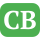CppBuzz.com

# C Programs which are not compiled with C++

We all know that C++ is designed to have backward compatibility with C programming but there can be many C programs that would produce compiler error when compiled with a C++ compiler. Following are few of them.

## 1) In C++, it is a compiler error to call a function before it is declared. But in C, it may compile

``````#include<stdio.h>

int main()
{
/* sum() is called before its declaration or definition */

sum(5, 10);
}

int sum(int a, int b)
{
printf(" sum is : %d", a+b);
return 0;
}```
```

## 2) In C++, it is compiler error to make a normal pointer to point a const variable, but it is allowed in C

``````#include <stdio.h>

int main(void)
{
int const a = 20;

/* The below assignment is invalid in C++, results in error
In C, the compiler *may* throw a warning, but casting is
implicitly allowed */

int *ptr = &a;  // A normal pointer points to const

printf("*ptr: %d\n", *ptr);

return 0;
}```
```

## 3) In C, a void pointer can directly be assigned to some other pointer like int *, char *

But in C++, a void pointer must be explicitly typcasted.

``````#include <stdio.h>
int main()
{
void *vptr;

/*In C++, it must be replaced with int *iptr=(int *)vptr;*/

int *iptr = vptr;

return 0;
}
``````

This is something we notice when we use malloc(). Return type of malloc() is void *. In C++, we must explicitly typecast return value of malloc() to appropriate type, e.g., “int *p = (void *)malloc(sizeof(int))”. In C, typecasting is not necessary.

## 4) Following program compiles & runs fine in C, but fails in compilation in C++.

const variable in C++ must be initialized but in c it isn’t necessary.

``````#include <stdio.h>
int main()
{
const int a; // LINE 4
return 0;
}
``````

Line 4 [Error] uninitialized const 'a' [-fpermissive]

## 5) This is the worst answer among all, but still a valid answer. We can use one of the C++ specific keywords as variable names.

The program won’t compile in C++, but would compiler in C.

``````#include <stdio.h>
int main(void)
{
/*new is a keyword in C++, but not in C*/

int new = 5;
printf("%d", new);

}``````

Similarly, we can use other keywords like delete, explicit, class, .. etc.

## 6) C++ does more strict type checking than C.

For example the following program compiles in C, but not in C++. In C++, we get compiler error invalid conversion from ‘int’ to ‘char*'

``````
#include <stdio.h>
int main()
{
char *c = 333;
printf("c = %u", c);
return 0;
}
``````